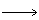# Chemistry Assignment Help with Order of a Reaction

## 4.3 Order of a Reaction

The rate of a chemical reaction is proportional to the product of effective concentration (acting masses) of the reacting species, each raised to a power that is equal to the corresponding stoichiometric number of the substance appearing in the chemical reaction.

Thus, for a general reaction aA + bBcC + dD …(2a)

We have

r = k[A]a [B]b …(2b)where k is the constant of proportionality.

If the rate of a reaction is determined experimentally, it is found that equation (2b) is not always applicable. However, the experimental results can be fitted to satisfy a relation of the type of equation (2b) where the exponents may or may not be equal to the respective stoichiometry coefficients. In general, we may write the rate as

R = k [A]a¢ [B]b¢ …(2c)

where the dimensionless exponents a¢ & b¢ may or may not be equal to a & b respectively.

The constant k, which appears in equation (2c) is known as rate constant or more formally, the specific reaction rate constant, since it is numerically equal to the rate of the reaction provided all concentration were set equal to unity. It may be emphasized once again here that the rate equation with its rate constant and order of various reacting species is an experimental finding and cannot be predicted from the stoichiometry of the balanced reaction.### Email Based Assignment Help in Order of a Reaction

We are the leading online Assignment Help provider. Find answers to all of your doubts regarding the Order of a Reaction in chemistry. We at assignmenthelp.net provide homework, Assignment Help to the school, college or university level students. Our expert online tutors are available to help you in Order of a Reaction. Our service is focused on: time delivery, superior quality, creativity and originality.